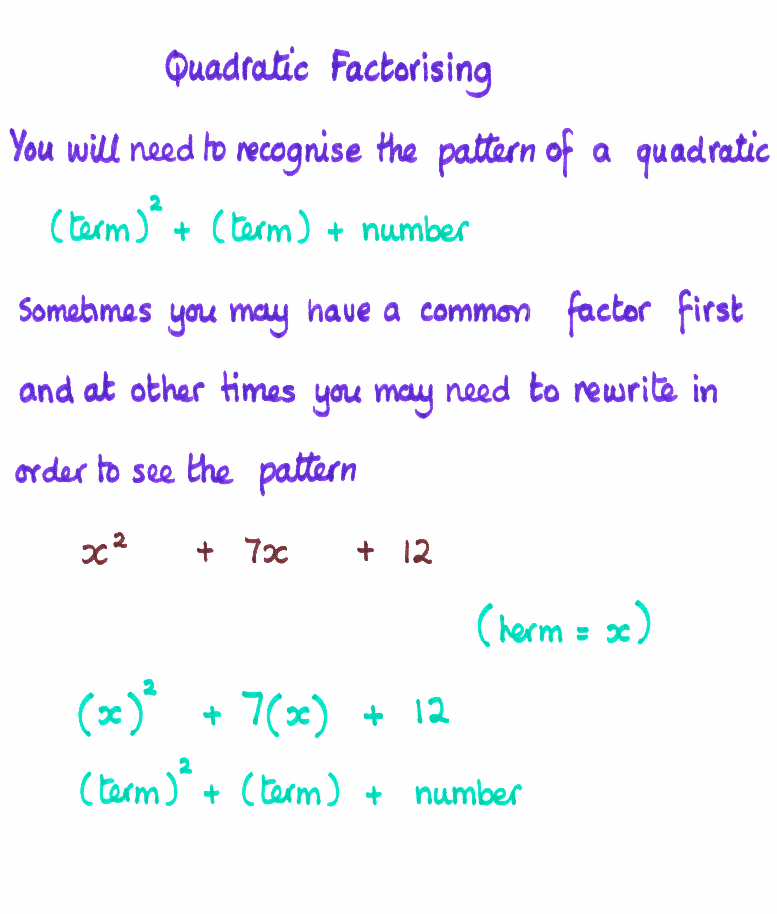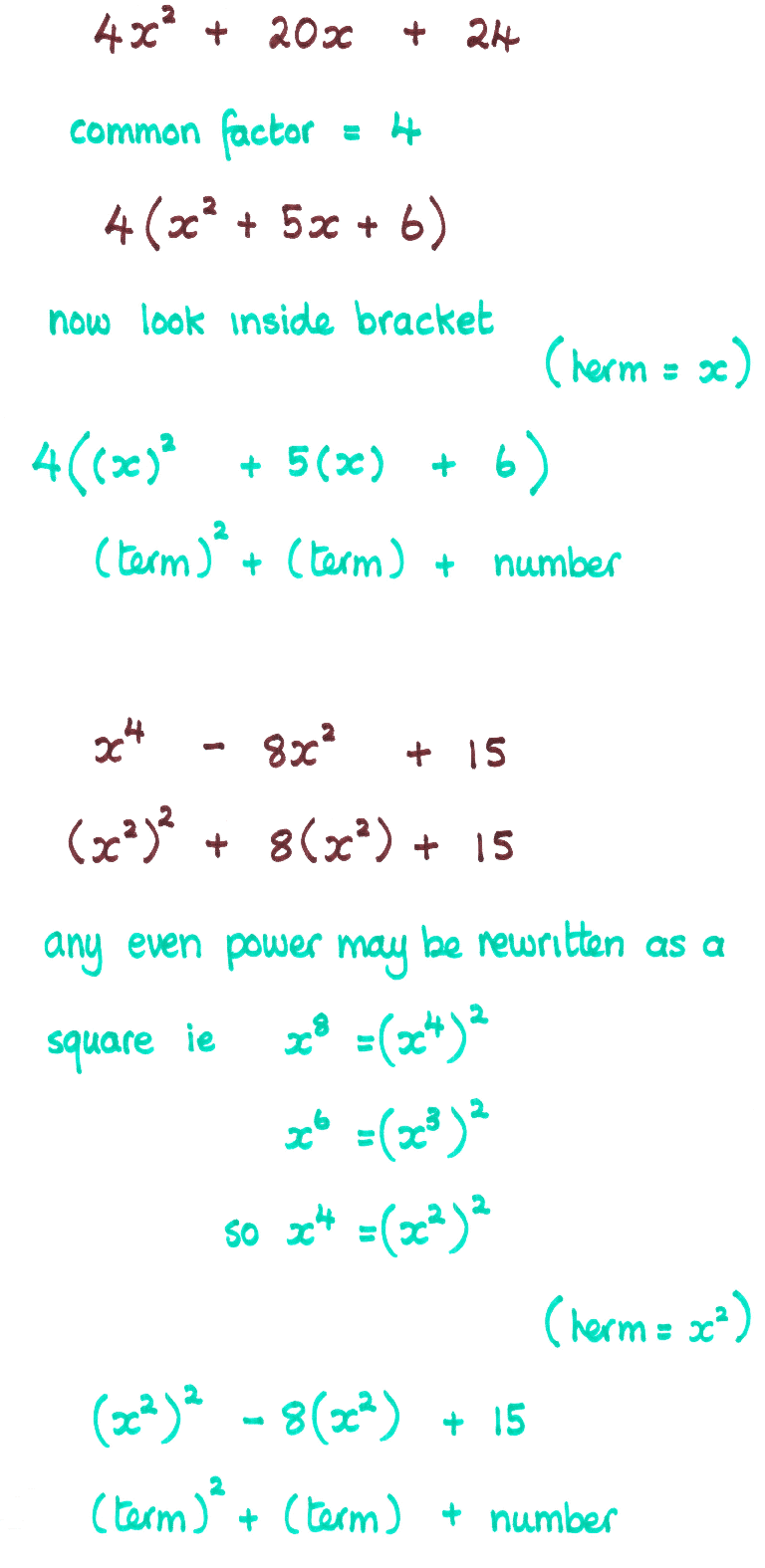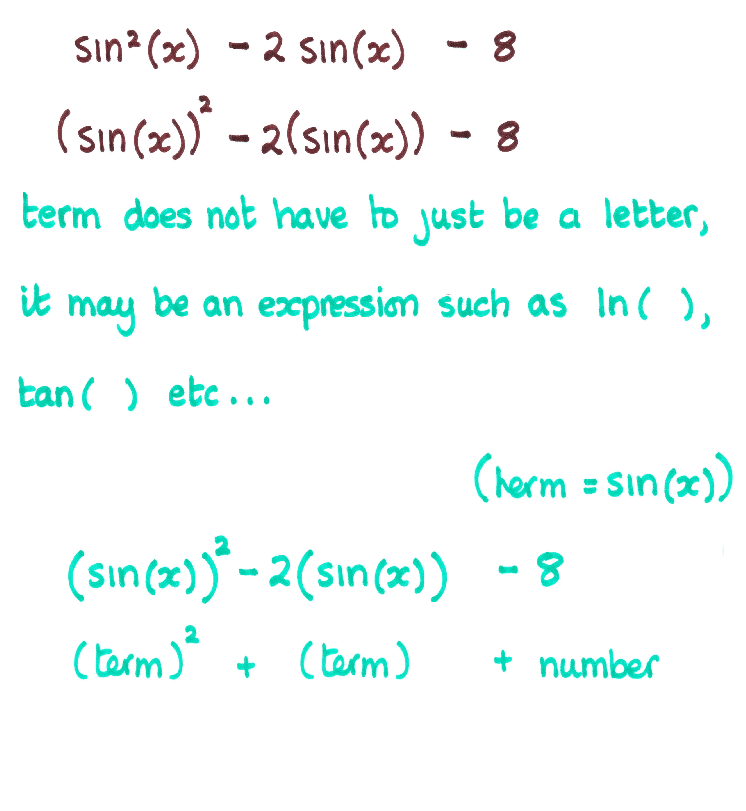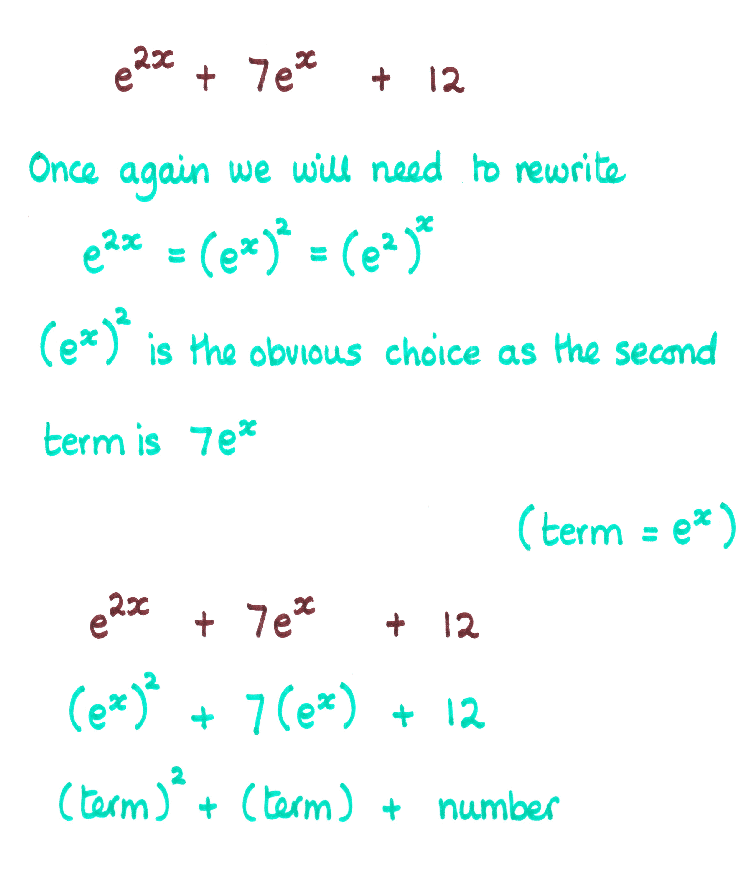Coming to Statistica
in 2022/2023

Group Classes

Interactive Webinars

Blog

Guestbook

.

E-mail        anne@statistica.com.au

# Place         Willetton, Western Australia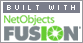# Mathematics & Statistic Tutor Perth - SPSS HelpThis type of factorising really needs to have someone there in person taking you through each step.  Alas I cannot do that unless you all pay for my air ticket!
What I am going to try to do is write down exactly what I would say and use lots of colours where I would have done actions ie throwing my hands around etc..To begin our journey, lets start with an example of multiplying brackets together and see the pattern.  Hopefully this will help us understand how to factorise a quadratic.         (x  +  4)( x  +  5)  I like to have a routine to follow so I always multiply my brackets out the same way. Multiply the first term in the first bracket by all the terms in the second bracket.  Multiply the second term in the first bracket by all the terms in the second bracket.   Add/subtract all  these terms. Watch out for those minus signs!  Continue until the last term in the first bracket.(x  +  4)( x  +  5) Multiply the first term in the first bracket by all the terms in the second bracket.(x  +  4)( x  +  5) = (x  x   x)  +  (x   x  5)  there is more to come, this is just step one! Multiply the second term in the first bracket by all the terms in the second bracket.(x  +  4)( x  +  5) =  (x   x   x)  +  (x   x  5)  + (4   x   x) +   (4  x  5)  Stop as the first bracket only has two terms.  Notice we add or subtract all of these terms as appropriate. (x  +  4)( x  +  5) =  x2    +  5 x   + 4 x  +  4  x 5                                   =   x2    +  9 x    +   20